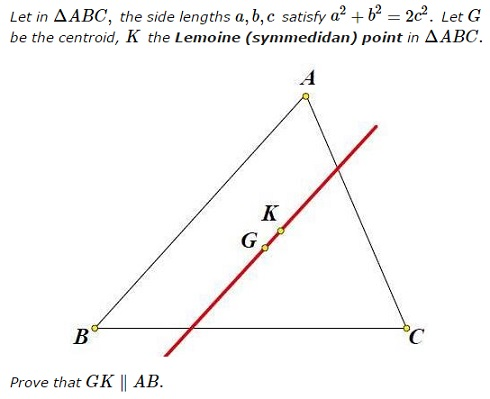# A Special Triangle with a Line Through the Lemoine Point

### Problem### Proof 1

Let $a^2=x,\,b^2=y,\,c^2=z.\,$ We have $x+y=2z.\,$ We know that

$\displaystyle\overrightarrow{AK}=\frac{y\overrightarrow{AB}+z\overrightarrow{AC}}{x+y+z}=\frac{y\overrightarrow{AB}+z\overrightarrow{AC}}{3z}.$

Also, $\displaystyle\overrightarrow{AG}=\frac{\overrightarrow{AB}+\overrightarrow{AC}}{3},\,$ implying $\overrightarrow{GK}=\overrightarrow{AK}-\overrightarrow{AG}=\displaystyle\frac{(y-z)\overrightarrow{AB}}{3z},\,$ so that, indeed, $GK\parallel AB.$

### Proof 2

In homogeneous barycentric coordinates, $G=1:1:1\,$ while $K=a^2:b^2:c^2.\,$ The equation of the side line $AB\,$ is $z=0.\,$ The equation of line $GK\,$ is

$\left|\begin{array}{ccc}\,x&y&z\\1&1&1\\a^2&b^2&c^2\end{array}\right|=(c^2-b^2)x+(a^2-c^2)y+(b^2-a^2)z=0.$

Since $c=\displaystyle\frac{a^2+b^2}{2},\,$ the equation of $GK\,$ is

$(a^2-b^2)x+(a^2-b^2)y+(b^2-a^2)z=0.$

The intersection of $GK\,$ with the line at infinity $x+y+z=0\,$ is $1:-1:0.\,$ This point also lies on $z=0,\,$ implying that the two lines intersect on the line at infinity. Hence, they are parallel.

### Acknowledgment

Leo Giugiuc has kindly posted at the CutTheKnotMath facebook page the above problem, due to Kadir Altintas, with his solution (Proof 1) and the solution by the problem's author (Proof 2).

### Symmedian